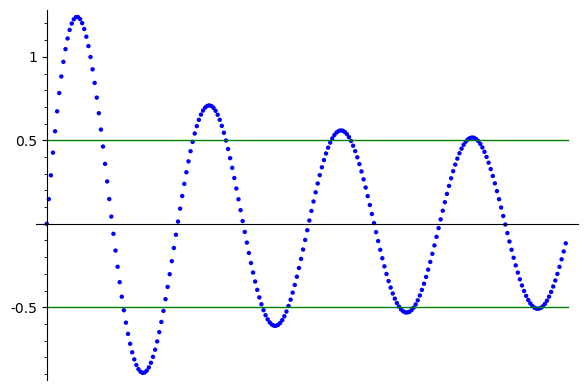All this to say I’ve started reading more measure theory books, though it makes my pile of unread logic and algebra books sad. In particular I bought a copy of Halmos from an online used bookstore for $8 (!!), and it’s a fantastic read so far. I wanted to highlight one particular observation that, while obvious in hindsight, was extremely helpful for me. Hopefully by posting about it here, I’ll also find it more memorable. While this isn’t a “trick” in the same sense as the other posts in this series, I still feel like it fits here. If$(E_n)$is a sequence of sets, then •$\limsup E_n$is the set of$x$that appear in infinitely many$E_n$•$\liminf E_n$is the set of$x$that appear in all but finitely many$E_n$I think I didn’t see this because I was too focused in on generalizing my understanding of$\liminf$and$\limsup$of sequences$(x_n)$. In the case of real sequences,$\liminf$is the smallest thing some subsequence converges to, while$\limsup$is the biggest thing some subsequence converges to. I think the following image does a good job showing what I mean:If this is your sequence$x_n$, then the biggest thing you can possibly converge to is$\frac{1}{2}$. Similarly, the smallest thing you can possibly converge to is$-\frac{1}{2}$. So these are$\limsup x_n$and$\liminf x_n$respectively. From this point of view it is clear that$\liminf x_n \leq \limsup x_n$, and whenever they are equal,$\lim x_n$exists and agrees with them both. Here$\liminf x_n = \lim_{n \to \infty} \inf_{m > n} x_m$, and dually,$\limsup x_n = \lim_{n \to \infty} \sup_{m > n} x_m$. This, of course, is where the notation comes from, but I think a better definition is •$\limsup x_n = \inf_n \sup_{m > n} x_m$•$\liminf x_n = \sup_n \inf_{m > n} x_m$Not only does this make it much more obvious that these definitions are dual, it more readily generalizes to the definition for sets: •$\limsup E_n = \bigcap_n \bigcup_{m > n} E_m$•$\liminf E_n = \bigcup_n \bigcap_{m > n} E_m$(As a quick check in – why are the two definitions of$\liminf x_n$equivalent?) Because I liked my intuition for$\limsup$and$\liminf$of sequences of reals, I’d been viewing$\liminf$and$\limsup$of sets as “the smallest (resp. largest) set that$E_n$could converge to”… Of course, I have no intuition for what it means for a sequence of sets to converge! Because of this, until today I’ve had little to no intuition for what these sets actually are. As with all realizations, I should have seen this much sooner. It’s a common trick in descriptive set theory to pass between logical constructors and set theoretic operations. These are entirely natural, and correspond to a correspondence between the (syntactic) boolean algebra of propositions, and the (semantic) boolean algebra of sets. Yet again I’m talking about syntax and semantics on this blog, and yet again I’m promising a post detailing a few of my favorite examples. For now though, let’s write this one example out explicitly: Say we have a family of properties$P_n$. Then •$\{ x \mid P_0 \land P_1 \} = \{ x \mid P_0 \} \cap \{ x \mid P_1 \}$(conjunction corresponds to intersection) •$\{ x \mid P_0 \lor P_1 \} = \{ x \mid P_0 \} \cup \{ x \mid P_1 \}$(disjunction corresponds to union) •$\{ x \mid \lnot P_0 \} = \{ x \mid P_0 \}^c$(negation corresponds to complementation) •$\{ x \mid T \} = X$(“true” corresponds to the whole set) •$\{ x \mid F \} = \emptyset$(“false” corresponds to the empty set) Quantifiers might seem tricky at first, but notice$\forall n . P_n$is really the same thing as$P_0 \land P_1 \land P_2 \land \ldots$and so: •$\{ x \mid \forall n . P_n \} = \bigcap_n \{ x \mid P_n \}$•$\{ x \mid \exists n . P_n \} = \bigcup_n \{ x \mid P_n \}$This trick works more broadly too1. For any index set$I$, we have •$\{ x \mid \forall \alpha \in I . P_\alpha \} = \bigcap_I \{ x \mid P_\alpha \}$•$\{ x \mid \exists \alpha \in I . P_\alpha \} = \bigcup_I \{ x \mid P_\alpha \}$Since we only know that countable setwise operations are allowed in measure theory, much of descriptive set theory amounts to showing that certain quantifiers only need to range over countable sets. Of course, through this lens, the description in Halmos is obvious: $\limsup E_n = \bigcap_n \bigcup_{m > n} E_n = \{ x \mid \forall n . \exists m > n . x \in E_n \} = \{ x \mid \text{x \in E_n for infinitely many n} \}$ Do you see why this also shows that$\liminf E_n = \{ x \mid \text{ $x$ is in all but finitely many $E_n$ } \}$? This viewpoint is useful not only in understanding what$\limsup E_n$and$\liminf E_n$are, it’s useful in proving things about them! Let$E_n$be a sequence that alternates between two sets$A$and$B$. Is it obvious that$\limsup E_n = A \cup B$and$\liminf E_n = A \cap B$? Now look only at the definitions – is it only obvious from those? I think this lens sheds some light on$\liminf$and$\limsup$of real sequences too. I’ll leave it to you to work through the quantifiers, but it turns out that$\limsup x_n$is the smallest number$x^*$so that infinitely many$x_n$are bigger than$x^*$. This is the central observation in the proof of the Cauchy-Hadamard Theorem, and it’s nice to see that this observation is actually obvious (in this interpretation of$\limsup$). Dually,$\liminf x_n$is the biggest number$x_*$so that infinitely many$x_n$are smaller than$x_*$. As a last puzzle – why doesn’t$\liminf x_n$have a “all but finitely many” flavor like$\liminf E_n$does? Can you find a sense in which it does? 1. It turns out viewing quantifiers as generalized conjunctions/disjunctions works very broadly! This is a useful viewpoint to take in many settings throughout logic. In$\mathcal{L}_{\omega_1, \omega}\$ for instance, this trick lets us use natural number quantifiers (even though the language might not technically allow them). This lets us express, say, that a group is finitely generated by writing every element as a word in the generators (and there’s only countably many such words!)